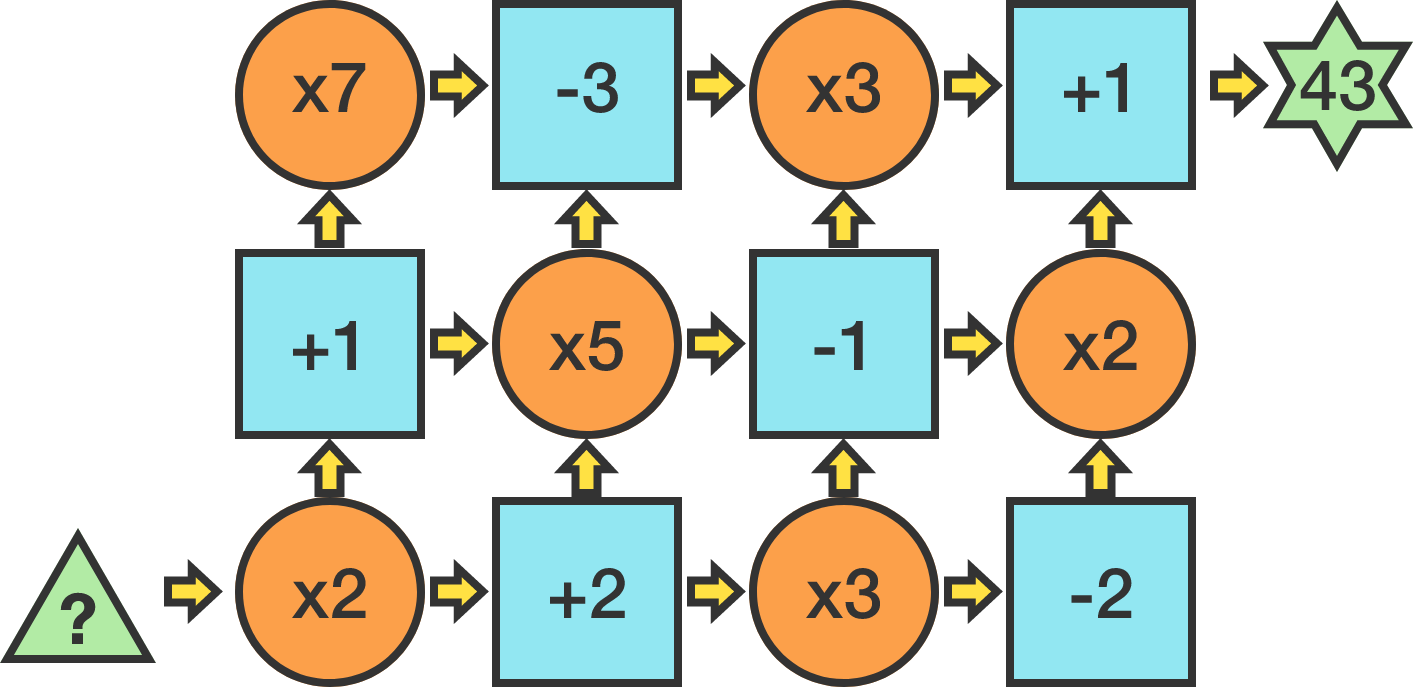# Number Factory

Logic Level 1The calculator above takes in an integer input at the lower left. Then, a path is taken to the top right - at each step moving up or right - and the operations given are performed on the input sequentially.

If a path gives an output of 43, which of the following choices could have been the initial input?

×